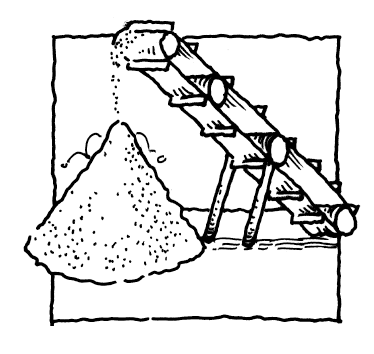### Home > APCALC > Chapter 7 > Lesson 7.2.3 > Problem7-79

7-79.

Grain pouring from a conveyor belt falls into a pile in the shape of a cone. The grain is falling at a rate of $8 \ \text{ m}^3\text{/min}$ and forms a pile such that the radius is always half the height. How fast is the height of the pile increasing when the radius is $6$ meters?

Sketch the cone, and label the sides of similar right triangles that are formed by its radius and height.

Write a geometric equation describing the volume of the cone in terms of height.
You will have to find a way to define $r$ in terms of $h$.
Since the radius changes as the height changes, do NOT 'plug in' any values yet.

Implicitly differentiate with respect to time, $t$.

$\text{'Plug in' the given values and solve for }\frac{d\text{(height)}}{dt}.$